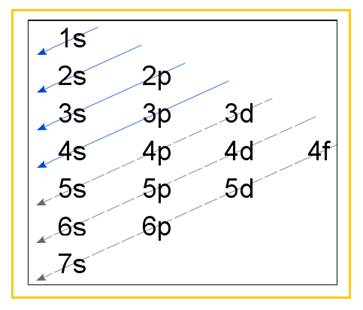Chapter 21, Problem 22E

Chapter
Section
Textbook Problem

# Write electron configurations for the following ions.  a.    Ni2+  b.    Cd2+  c.    Zr3+ and Zr4+  d.    Os2+ and Os3+

(a)

Interpretation Introduction

Interpretation:

Electronic configuration of the following metals has to be written –

(a)   Ni2+(b) Cd2+(c) Zr3+, Zr4+(d) Os2+, Os3+

Concept Introduction:

Electronic configuration of an atom represents the arrangement of electrons in various energy levels.  The electrons are arranged in increasing order of energy levels according to Aufbau principle.  It is pictorially represented as –Figure 1

The terms 1s, 2s, 3s ... etc represent the energy levels inside an atom in which electrons are filled.

Explanation

Atomic number of Nickel is 28 . Accordingly the electronic configuration is –

1s22s22p63s23p63d84s2

Ni2+ is formed when Nickel loses two electrons

(b)

Interpretation Introduction

Interpretation:

Electronic configuration of the following metals has to be written –

(a)   Ni2+(b) Cd2+(c) Zr3+, Zr4+(d) Os2+, Os3+

Concept Introduction:

Electronic configuration of an atom represents the arrangement of electrons in various energy levels.  The electrons are arranged in increasing order of energy levels according to Aufbau principle.  It is pictorially represented as –Figure 1

The terms 1s, 2s, 3s ... etc represent the energy levels inside an atom in which electrons are filled.

(c)

Interpretation Introduction

Interpretation:

Electronic configuration of the following metals has to be written –

(a)   Ni2+(b) Cd2+(c) Zr3+, Zr4+(d) Os2+, Os3+

Concept Introduction:

Electronic configuration of an atom represents the arrangement of electrons in various energy levels.  The electrons are arranged in increasing order of energy levels according to Aufbau principle.  It is pictorially represented as –Figure 1

The terms 1s, 2s, 3s ... etc represent the energy levels inside an atom in which electrons are filled.

(d)

Interpretation Introduction

Interpretation:

Electronic configuration of the following metals has to be written –

(a)   Ni2+(b) Cd2+(c) Zr3+, Zr4+(d) Os2+, Os3+

Concept Introduction:

Electronic configuration of an atom represents the arrangement of electrons in various energy levels.  The electrons are arranged in increasing order of energy levels according to Aufbau principle.  It is pictorially represented as –Figure 1

The terms 1s, 2s, 3s ... etc represent the energy levels inside an atom in which electrons are filled.

### Still sussing out bartleby?

Check out a sample textbook solution.

See a sample solution

#### The Solution to Your Study Problems

Bartleby provides explanations to thousands of textbook problems written by our experts, many with advanced degrees!

Get Started